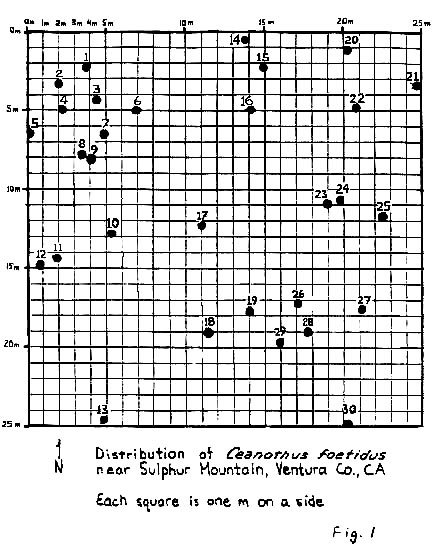# Biogeography

### Lab 1: Nearest-Neighbor AnalysisOne of the things a biogeographer might want to do is evaluate the spatial pattern of a plant species' distribution in a particular area. There are all kinds of techniques that can be used for this sort of "point pattern analysis" problem. This lab introduces you to one technique in biogeography, nearest- neighbor analysis, which is often used to describe a spatial pattern as "clumped," "random," or "uniform."

For all questions on this lab, please do your calculations at the full capacity of your spreadsheet or calculator, but round your answers at the very end to three decimal places of accuracy (i.e., 0.000).### Lab 1a: Basic Data Needed for Nearest-Neighbor Analysis

Examine the distribution of adult fetid lilac shrubs, Ceanothus foetidus (which I made up, by the way, so don't go running to the Munz flora in the excitement of learning about a new chaparral plant!) in Figure 1. The area mapped is a hillside in the Sulphur Mountain area of Ventura County (which does exist and is well named!). In your cursory look at this map, do you see a tendency for the plants to cluster at all, or do they seem uniform (like an orchard) in their distribution?

You get to employ nearest-neighbor analysis to ascertain whether your hunch is right: Nearest-neighbor analysis creates a descriptive statistic, R, which indicates whether this "species" at this scale has a clumped, uniform, or random distribution.

Calculating the nearest-neighbor co-efficient (R) entails the tedious process of measuring the distance between each point in a given space and the point that is its nearest neighbor. It should be noted that Point A may well have Point B as its nearest neighbor, but Point B may have another point entirely, say, C, as its nearest neighbor. Anyhow, having measured all those nearest neighbor distances, you figure out the mean nearest-neighbor distance

Next, you then create an expected mean nearest-neighbor distance from the density of the points in your study area. You are now in a position to create R as the ratio of the mean observed nearest-neighbor distance to this expected mean nearest-neighbor distance.

R can vary from 0 to 2.149 (I've always liked that extra .149 bit!). A score of 0 means perfect clustering: All points are found at the exact same point in space (which is, of course, a physical impossibility if your study entails point data collected at one time). A score of 2.149 means perfect uniformity. A score of 1 represents perfectly random distribution of points in space. So, you can use this descriptive statistic to characterize a distribution as more clustered or more uniform or just random.

1. What is n (i.e., how many plants are there in the area shown in Figure 1)?
```     n = ________

```
2. What is A (the size of the study area, measured in square meters -- multiply the width of the study area in meters by the length of the study area in meters)?
```     A = ________

```
3. What is p (the density of C. foetidus in numbers per unit of area, or n/A, or, in this case, number of plants divided by the size of the study area in square meters)?
```     p = ________

```
4. The tedious part: Measure and record the distance from each plant to its nearest-neighbor (remember, one plant can wind up the nearest-neighbor for more than one other plant). The easiest way to do this is to print the map and then take a stiff piece of paper or card and align it somewhere on the grid. Mark the edge off in 1 m increments to create your own ruler for this lab (this saves you the extra work of measuring everything in inches or centimeters and then figuring out how many of these add up to 25 m and then mathematically converting the inches or centimeters into meters). Record your measurements (in meters) in the worksheet below. "#" stands for the individual plant identification number shown on the map, while "r" is the distance between each plant and its nearest neighbor.
```
#      r                 #      r                 #      r

1)  ________            11)  ________            21)  ________

2)  ________            12)  ________            22)  ________

3)  ________            13)  ________            23)  ________

4)  ________            14)  ________            24)  ________

5)  ________            15)  ________            25)  ________

6)  ________            16)  ________            26)  ________

7)  ________            17)  ________            27)  ________

8)  ________            18)  ________            28)  ________

9)  ________            19)  ________            29)  ________

10)  ________            20)  ________            30)  ________

```### Lab 1b: Calculating Ratio of Observed to Expected Nearest-Neighbor Distance

_
1. What is the ro (the observed mean nearest-neighbor distance)? This entails adding up all of those observed nearest-neighbor distances and then dividing them by the number of plants (30).
```     _
ro =  ________
```
_
2. What is the re (the expected mean nearest-neighbor distance)? In English, you divide n (30) by the A (study area). Then, you take the square root of your answer. Multiply that square root by 2. Now, divide 1 by THAT answer.
```     _           1                  1
re  =     ____       or       ___
____                ___
2\/ n/A             2\/  p

_
re  =  ________

```
3. What is R (the ratio of observed to expected mean nearest-neighbor distances)?
```             _
ro
R  =  _____
_
re

=  ________

```### Lab 1c: Brief Lab Report Interpreting Your Results

On the basis of the R you calculated and the guidelines for interpreting it given at the top of the lab, how would you characterize the local distribution of C. foetidus, at least at this particular scale of analysis? Considering your earlier hunch about the map, did the test results surprise you?
```     _________________________________________________________________________

_________________________________________________________________________

_________________________________________________________________________

_________________________________________________________________________

_________________________________________________________________________

_________________________________________________________________________

```first placed on the web: 11/26/98
last revised: 09/16/03